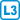## Monty Hall problem

On the game show Let's Make a Deal the host Monty Hall offers a prize under the following rules. The prize, a car, is hidden behind one of three doors. Each of the remaining doors hides a consolation prize – a goat. The player first points to any of the three doors. The host, who knows where the prize is hidden, opens one of the other doors, specifically the one that does not contain the prize. In this situation the player must now open one of the remaining two doors and will receive whatever is behind it.

Is it better for the player to change his mind and open the door other than the one he first pointed to?

• #### Hint

Assume without loss of generality that the player has pointed to the first door. Now consider the situation where the host has opened the second door, which we will call event B. For a correct decision you must determine the conditional probability $$P(A_i|B)$$, where $$A_i$$ indicates the event where the prize is behind the $$i$$-th door.

• #### Solution

First consider that we are working in a probability space $$(\Omega,P)$$ formed by nine elementary events $$(i,j)$$, where $$i$$ indicates the position of the prize and $$j$$ indicates the door opened by the host. For now we don't know the probabilities of the elementary events, with the exception of the cases $$i=j$$, which have probability zero.

To be able to use Bayes' theorem $$P(A_i|B)=\frac{P(A_i\cap B)}{P(B)}=\frac{P(A_i)P(B|A_i)}{P(B)}$$,

we must determine the probabilities $$P(A_i)$$, $$P(B|A_i)$$ and $$P(B)$$.

If the prize is positioned randomly, then $$P(A_i)=\frac13$$ for all three values of $$i$$.

By the nature of the game $$P(B|A_2)=0$$, since the host cannot open the door with the prize.

Similarly $$P(B|A_3)=1$$, because in this case the host has no choice.

On the other hand $$P(B|A_1)=\frac12$$, because the host can open either the second or third door.

To determine $$P(B)$$ we use the fact that the events $$A_i$$ are mutually exclusive and cover $$\Omega$$. So $$B=(B\cap A_1)\cup (B\cap A_2) \cup (B\cap A_3)$$, or $$P(B)=P(B\cap A_1)+P(B\cap A_2)+P(B\cap A_3)= P(A_1)P(B|A_1)+P(A_2)P(B|A_2)+P(A_3)P(B|A_3)=$$

$$\frac13\cdot\frac12 + \frac13{\cdot} 0+\frac13{\cdot} 1 = \frac12$$.

If the player sticks with his choice, the probability of winning the prize is: $$P(A_1|B)=\frac{\frac13\cdot\frac12}{\frac12}=\frac13$$.

If he changes his mind, his chance will be: $$P(A_3|B)=\frac{\frac13{\cdot}1}{\frac12}=\frac23$$.

Here is an alternative, less formal argument. The probability that the prize is behind the first door is $$\frac{1}{3}$$. The player will win the prize with this probability if he does not change his mind.

If he does change his mind, there is only one other door where the prize could be hidden. The probability of a win therefore equals the complement of the probability of a loss. He will lose if the prize is hidden behind the first door. As we have already calculated, that will happen with probability $$\frac{1}{3}$$, so the probability of a win is $$\frac{2}{3}$$.
It is better to change his mind, because in that case the player will win with probability $$\frac23$$.